# 你应该了解的25个JS技巧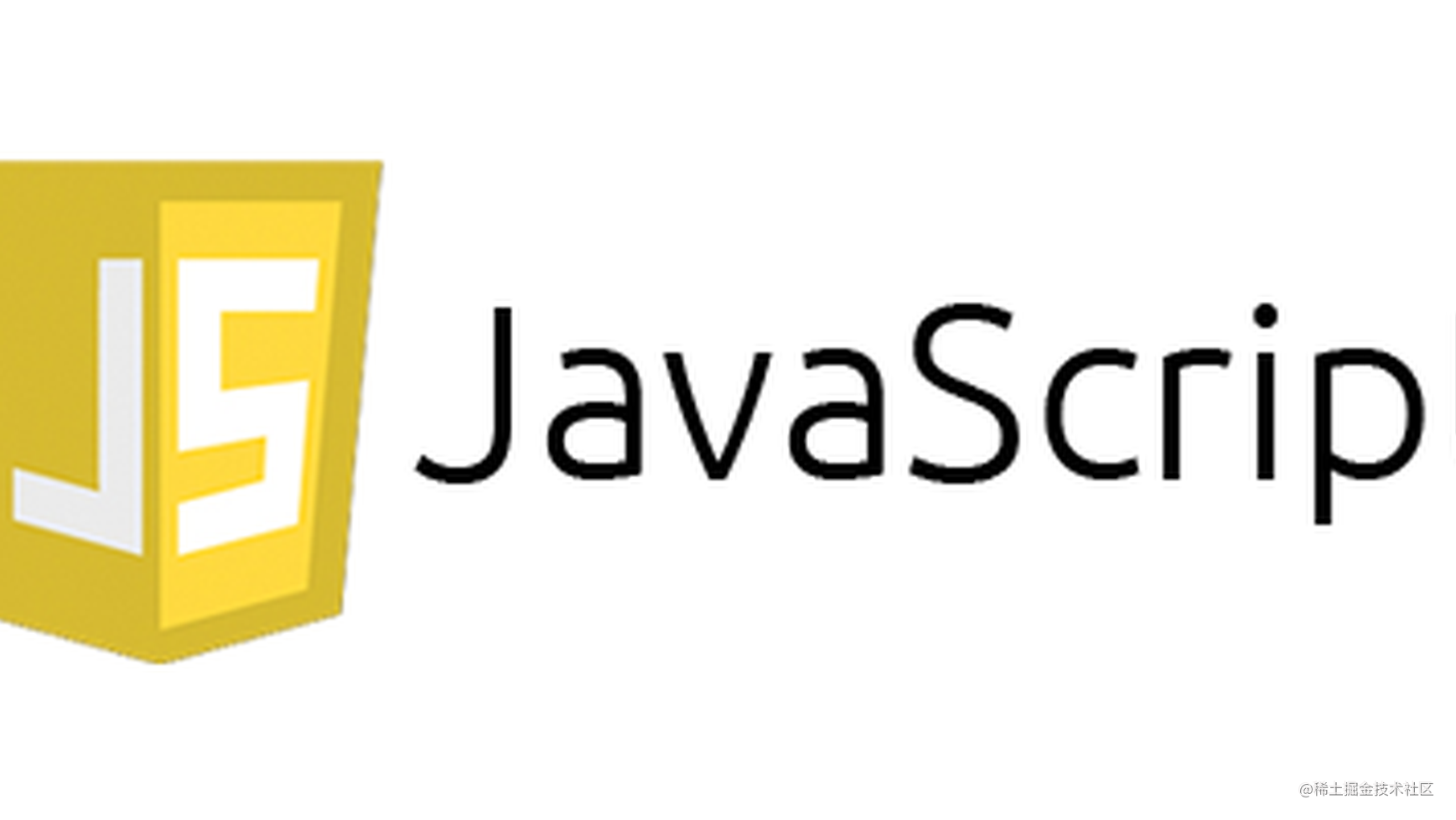## 1. 类型检查小工具

JavaScript 不是强类型语言，对此我推荐的最佳解决方案是 TypeScript。但有时你只是想要一个简单的类型检查，这种时候 JavaScript 允许你使用“typeof”关键字。

“typeof”的问题在于，将其用于某些原语和函数时效果很好，但对于数组和对象来说，由于它们都被视为“对象”，因此很难把握它们之间的区别。

``````const isOfType = (() => {
// create a plain object with no prototype
const type = Object.create(null);
// check for null type
type.null = x => x === null;
// check for undefined type
type.undefined = x => x === undefined;
// check for nil type. Either null or undefined
type.nil = x => type.null(x) || type.undefined(x);
// check for strings and string literal type. e.g: 's', "s", `str`, new String()
type.string = x => !type.nil(x) && (typeof x === 'string' || x instanceof String);
// check for number or number literal type. e.g: 12, 30.5, new Number()
type.number = x => !type.nil(x)
&& (// NaN & Infinity have typeof "number" and this excludes that
(!isNaN(x) && isFinite(x)
&& typeof x === 'number'
) || x instanceof Number);
// check for boolean or boolean literal type. e.g: true, false, new Boolean()
type.boolean = x => !type.nil(x) && (typeof x === 'boolean' || x instanceof Boolean);
// check for array type
type.array = x => !type.nil(x) && Array.isArray(x);
// check for object or object literal type. e.g: {}, new Object(), Object.create(null)
type.object = x => ({}).toString.call(x) === '[object Object]';
// check for provided type instance
type.type = (x, X) => !type.nil(x) && x instanceof X;
// check for set type
type.set = x => type.type(x, Set);
// check for map type
type.map = x => type.type(x, Map);
// check for date type
type.date = x => type.type(x, Date);
return type;
})();

## 2. 检查是否为空

``````function isEmpty(x) {
if(Array.isArray(x)
|| typeof x === 'string'
|| x instanceof String
) {
return x.length === 0;
}
if(x instanceof Map || x instanceof Set) {
return x.size === 0;
}
if(({}).toString.call(x) === '[object Object]') {
return Object.keys(x).length === 0;
}
return false;
}

## 3. 获取列表最后一项

``````function lastItem(list) {
if(Array.isArray(list)) {
return list.slice(-1);
}
if(list instanceof Set) {
return Array.from(list).slice(-1);
}
if(list instanceof Map) {
return Array.from(list.values()).slice(-1);
}
}

## 4. 带有范围的随机数生成器

``````function randomNumber(max = 1, min = 0) {
if(min >= max) {
return max;
}
return Math.floor(Math.random() * (max - min) + min);
}

## 5. 随机 ID 生成器

``````// create unique id starting from current time in milliseconds
// incrementing it by 1 everytime requested
const uniqueId = (() => {
const id = (function*() {
let mil = new Date().getTime();
while (true)
yield mil += 1;
})();
return () => id.next().value;
})();
// create unique incrementing id starting from provided value or zero
// good for temporary things or things that id resets
const uniqueIncrementingId = ((lastId = 0) => {
const id = (function*() {
let numb = lastId;
while (true)
yield numb += 1;
})()
return (length = 12) => `\${id.next().value}`.padStart(length, '0');
})();
// create unique id from letters and numbers
const uniqueAlphaNumericId = (() => {
const heyStack = '0123456789abcdefghijklmnopqrstuvwxyz';
const randomInt = () => Math.floor(Math.random() * Math.floor(heyStack.length))
return (length = 24) => Array.from({length}, () => heyStack[randomInt()]).join('');
})();

## 6. 创建一个范围内的数字

Python 里我很喜欢的一个功能是 range 函数，而在 JavaScript 里我经常需要自己写这个功能。下面是一个简单的实现，非常适合 for…of 循环以及需要特定范围内数字的情况。

``````function range(maxOrStart, end = null, step = null) {
if(!end) {
return Array.from({length: maxOrStart}, (_, i) => i)
}
if(end <= maxOrStart) {
return [];
}
if(step !== null) {
return Array.from(
{length: Math.ceil(((end - maxOrStart) / step))},
(_, i) => (i * step) + maxOrStart    );
}
return Array.from(
{length: Math.ceil((end - maxOrStart))},
(_, i) => i + maxOrStart  );
}

## 7. 格式化 JSON 字符串，stringify 任何内容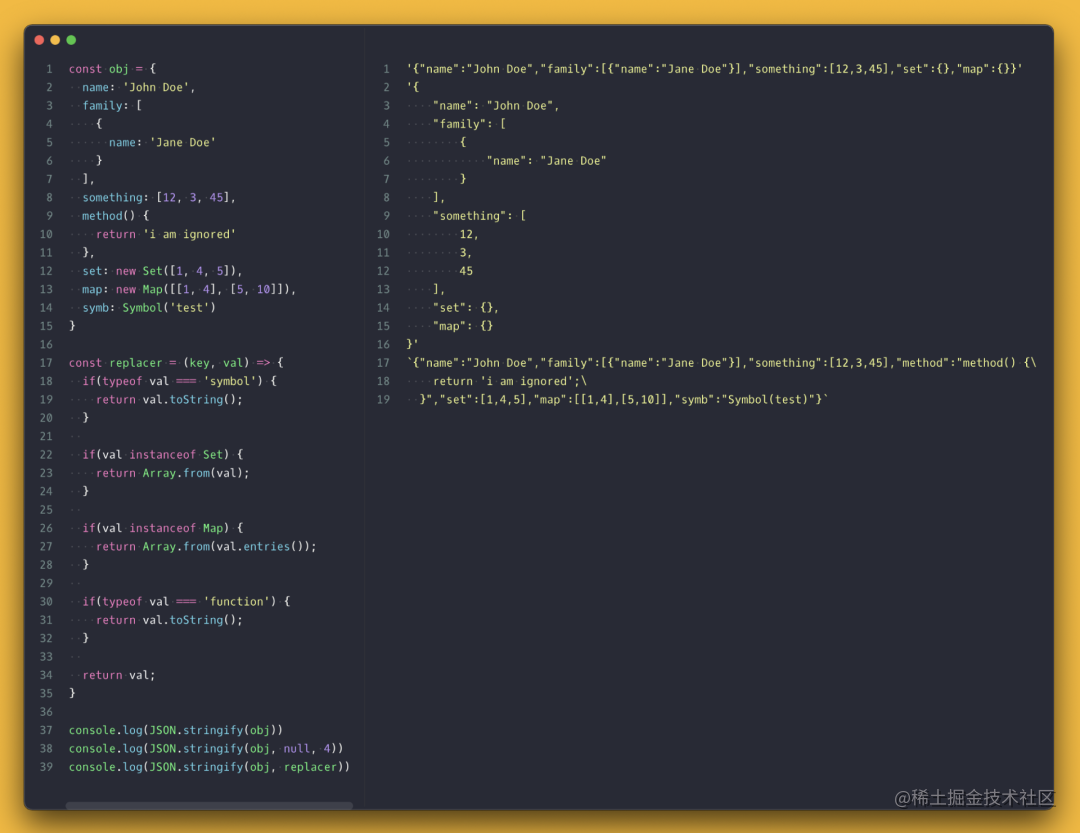``````const stringify = (() => {
const replacer = (key, val) => {
if(typeof val === 'symbol') {
return val.toString();
}
if(val instanceof Set) {
return Array.from(val);
}
if(val instanceof Map) {
return Array.from(val.entries());
}
if(typeof val === 'function') {
return val.toString();
}
return val;
}
return (obj, spaces = 0) => JSON.stringify(obj, replacer, spaces)
})();

## 8. 顺序执行 promise

``````const asyncSequentializer = (() => {
const toPromise = (x) => {
if(x instanceof Promise) { // if promise just return it
return x;
}
if(typeof x === 'function') {
// if function is not async this will turn its result into a promise
// if it is async this will await for the result
return (async () => await x())();
}
return Promise.resolve(x)
}
return (list) => {
const results = [];
return list
.reduce((lastPromise, currentPromise) => {
return lastPromise.then(res => {
results.push(res); // collect the results
});
}, toPromise(list.shift()))
// collect the final result and return the array of results as resolved promise
.then(res => Promise.resolve([...results, res]));
}
})();

## 9. 轮询数据

``````async function poll(fn, validate, interval = 2500) {
const resolver = async (resolve, reject) => {
try { // catch any error thrown by the "fn" function
const result = await fn(); // fn does not need to be asynchronous or return promise
// call validator to see if the data is at the state to stop the polling
const valid = validate(result);
if (valid === true) {
resolve(result);
} else if (valid === false) {
setTimeout(resolver, interval, resolve, reject);
} // if validator returns anything other than "true" or "false" it stops polling
} catch (e) {
reject(e);
}
};
return new Promise(resolver);
}

## 10. 等待所有 promise 完成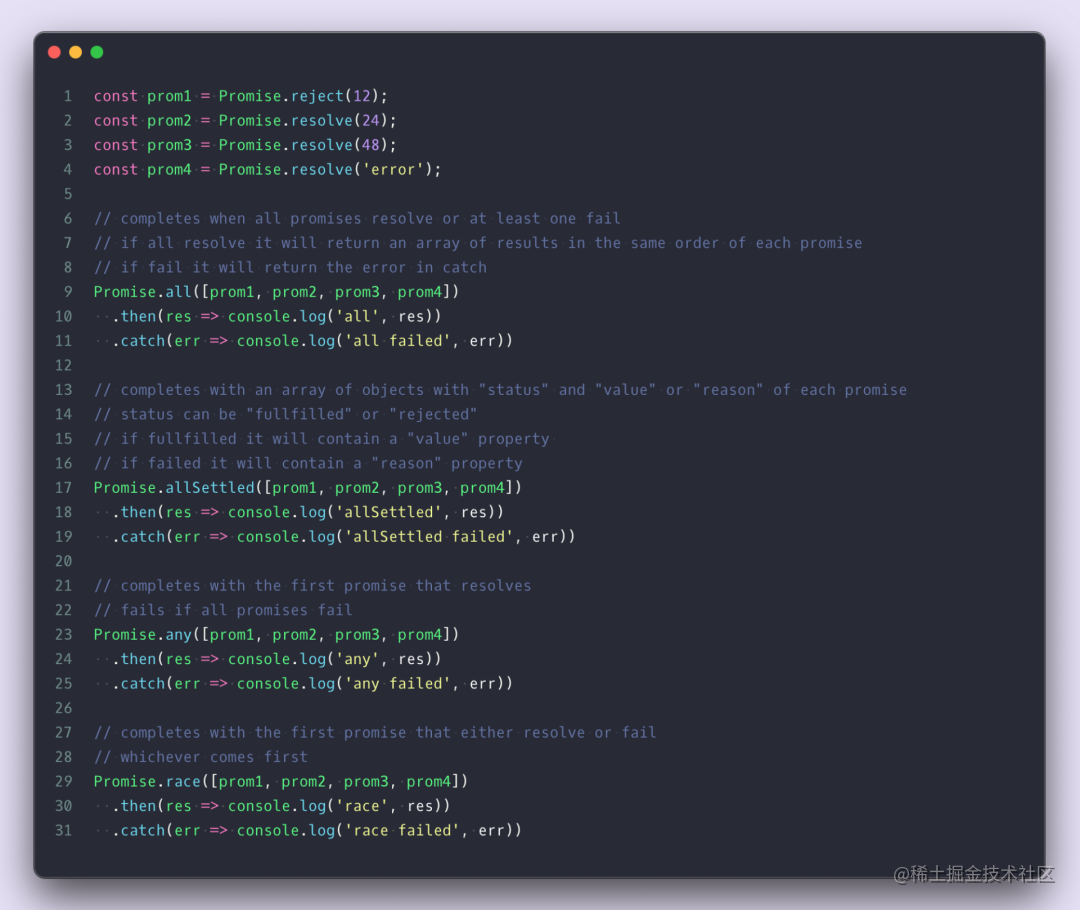## 11. 交换数组值的位置

ES6 开始，从数组中的不同位置交换值变得容易多了。这个做起来不难，但是了解一下也不错，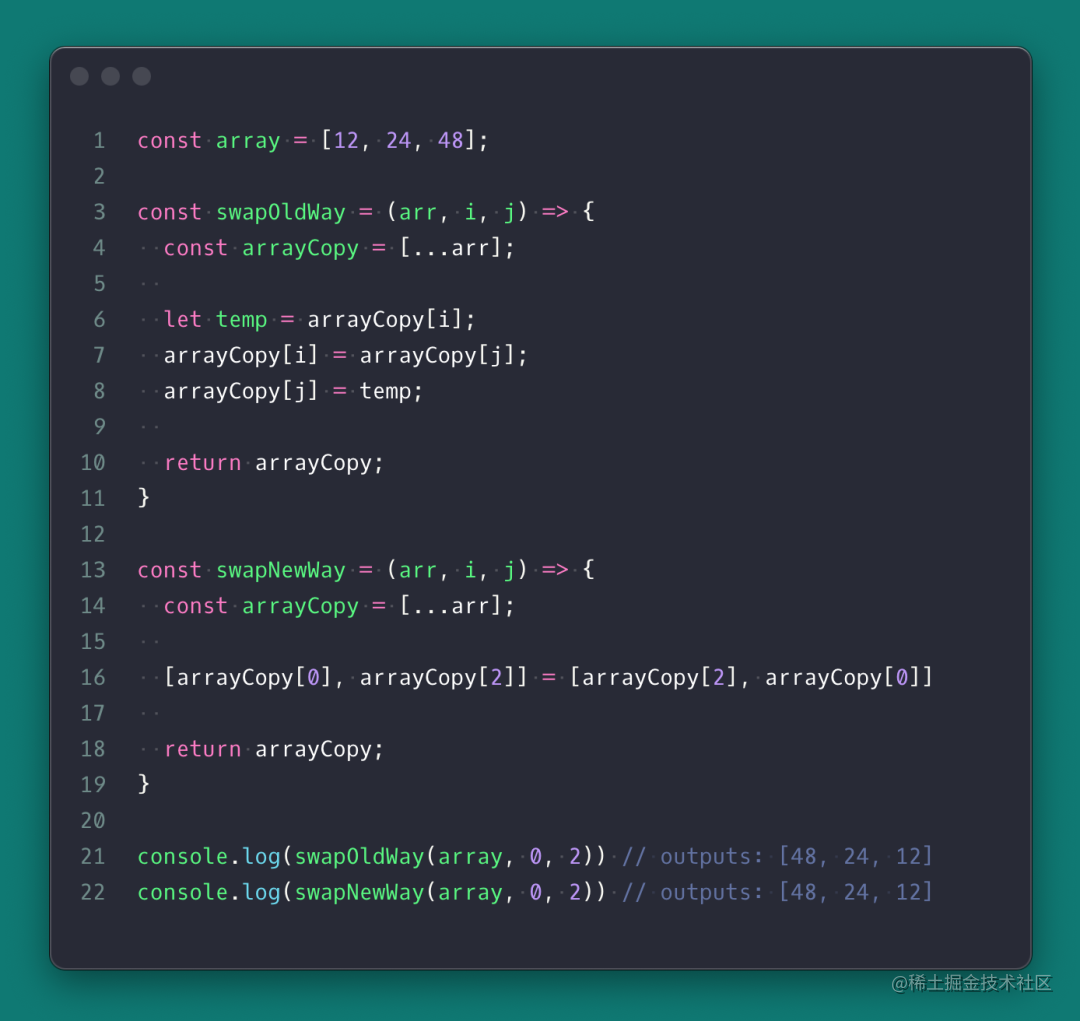## 12. 条件对象键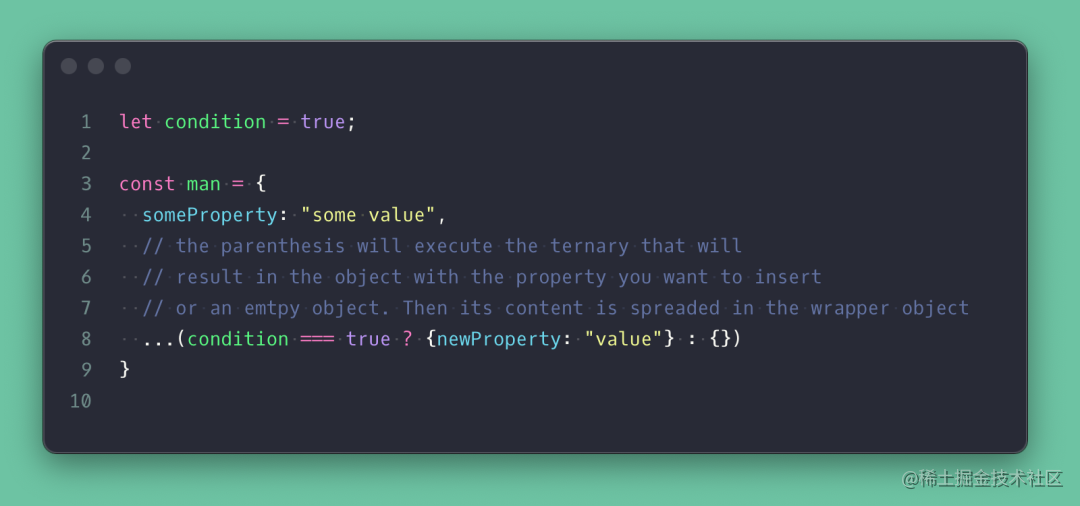## 13. 使用变量作为对象键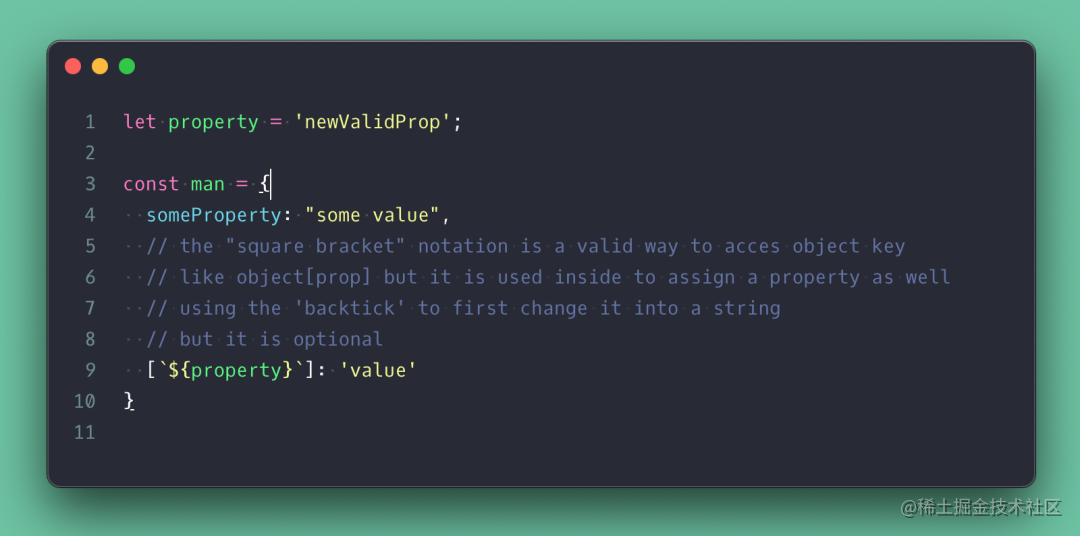## 14. 检查对象里的键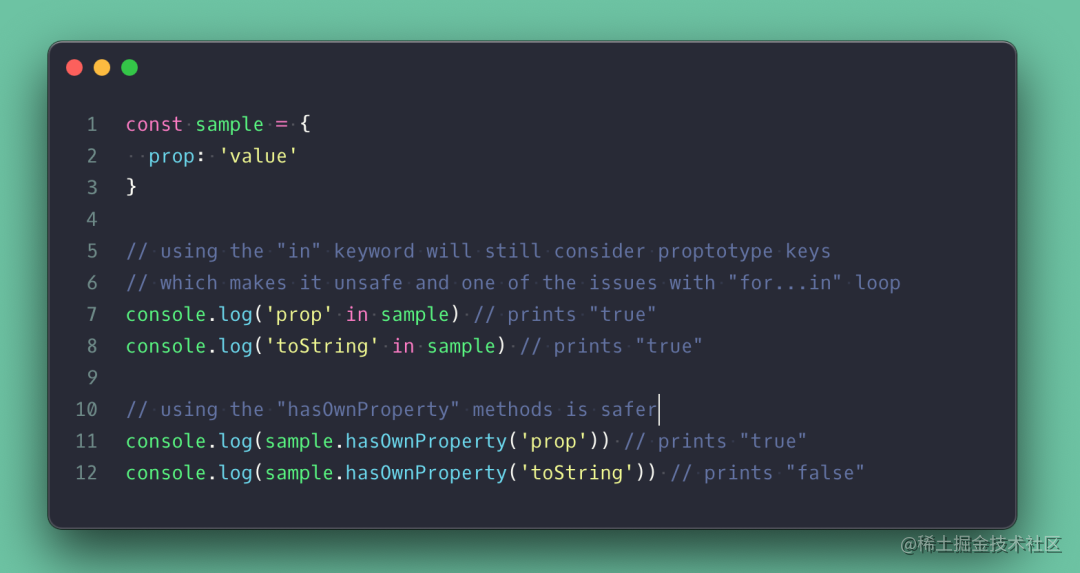## 15. 删除数组重复项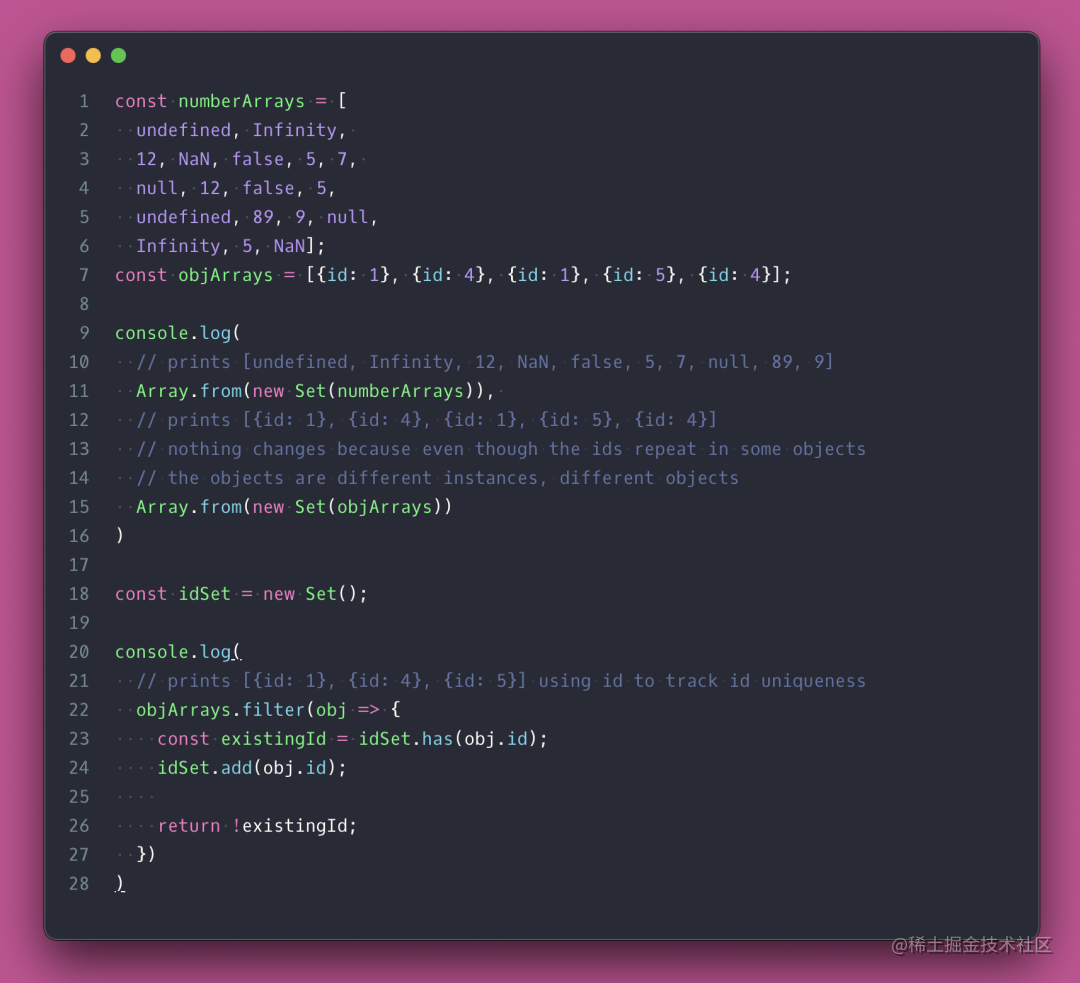## 16. 在 ArrayforEach 中执行“break”和“continue”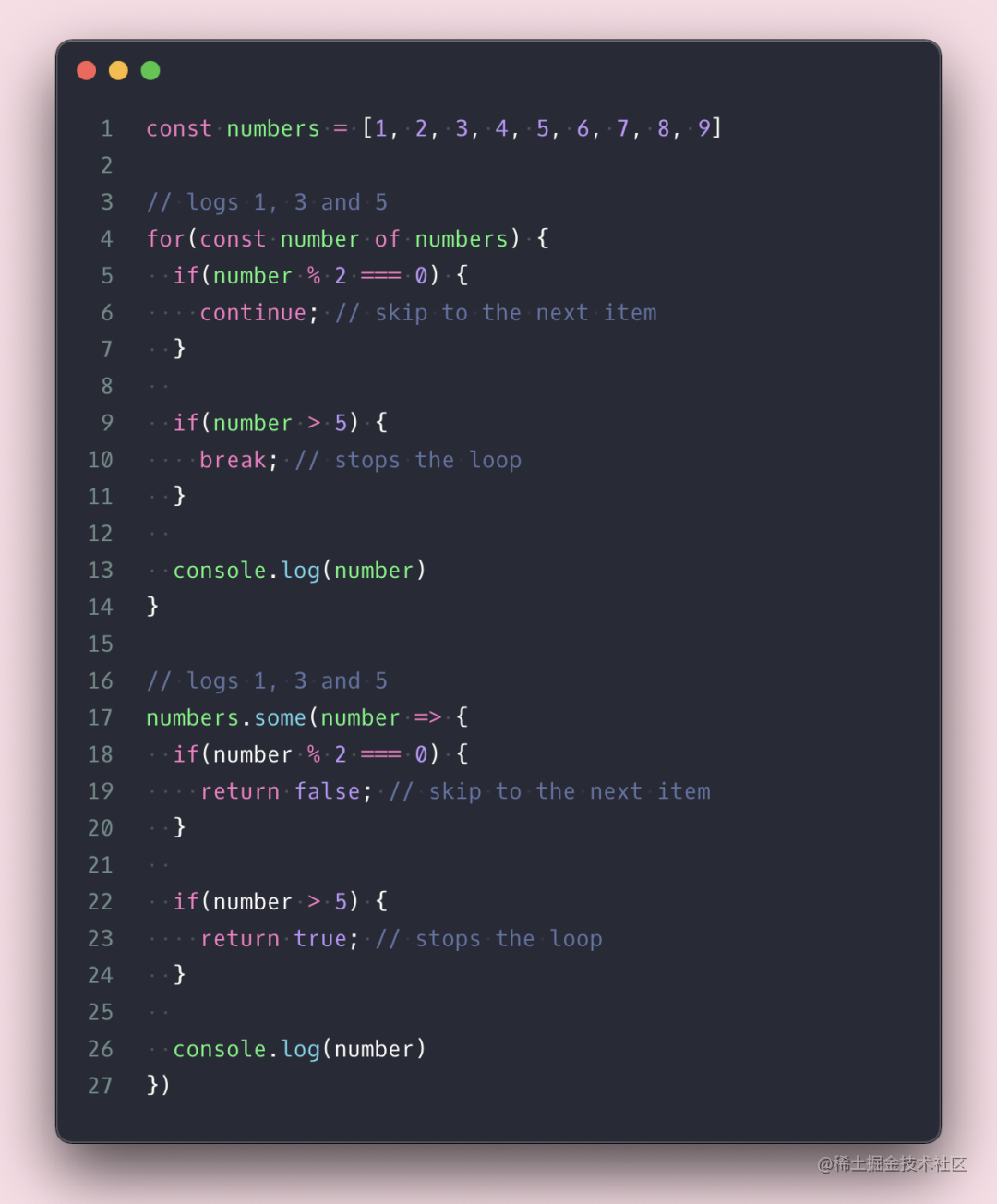## 17. 使用别名和默认值来销毁

Destructuring（销毁）是 JavaScript 最好用的功能之一，而且你可以使用“冒号”设置别名，并使用“等号”设置属性默认值。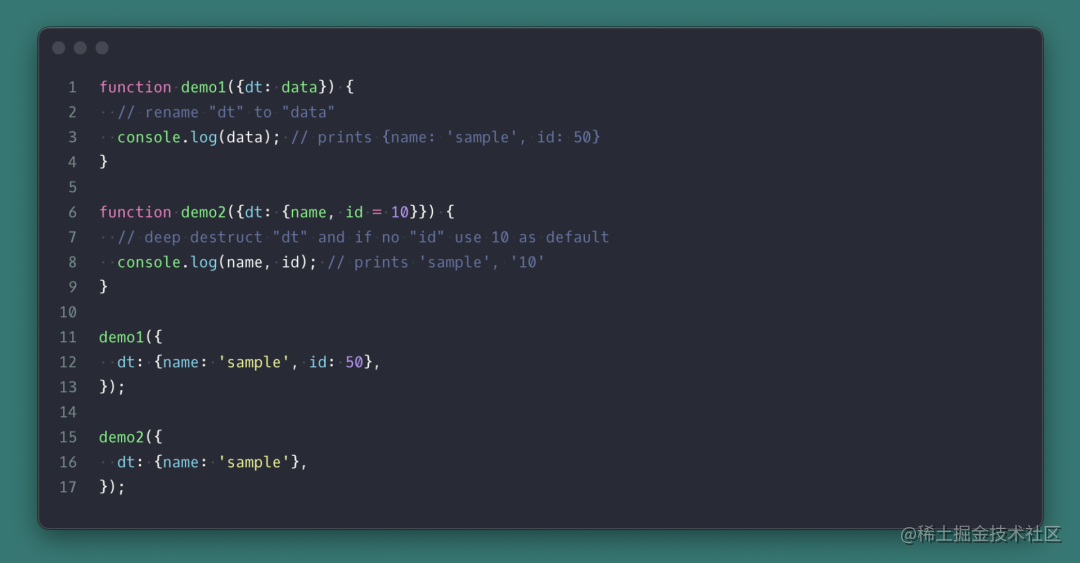## 18. 可选链和空值合并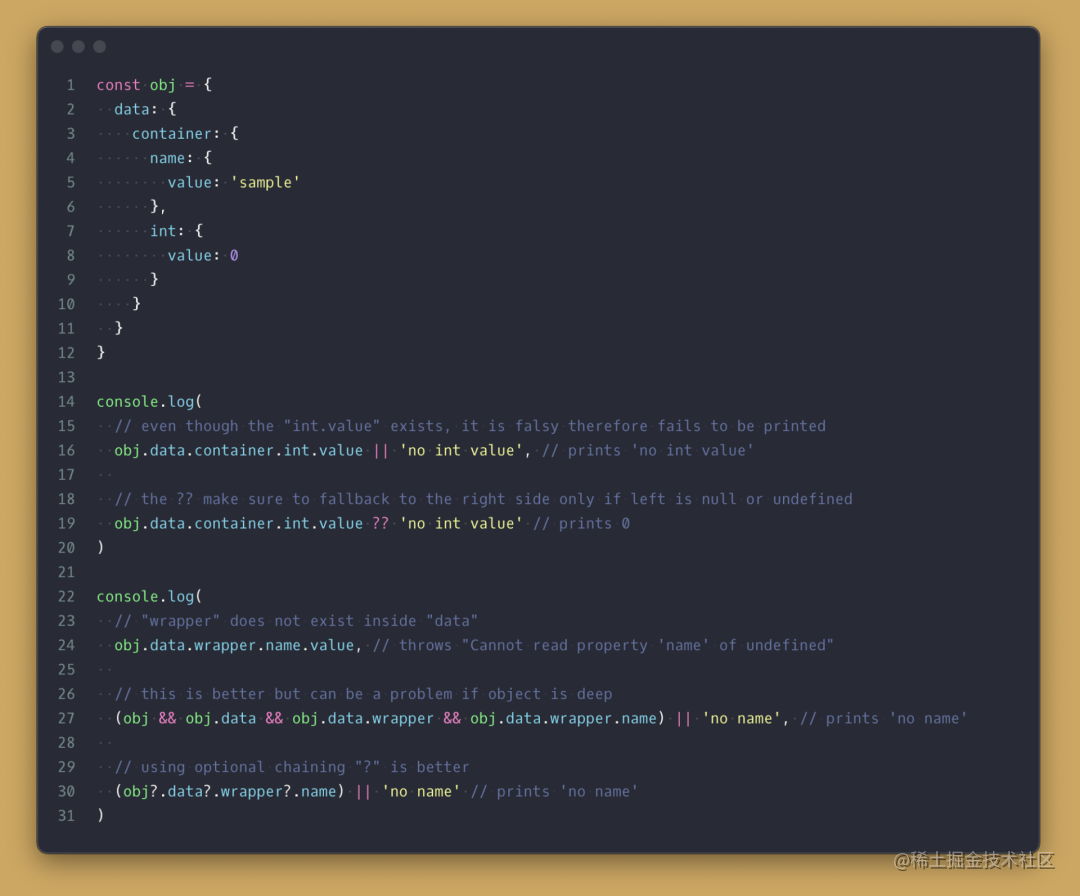## 19. 用函数扩展类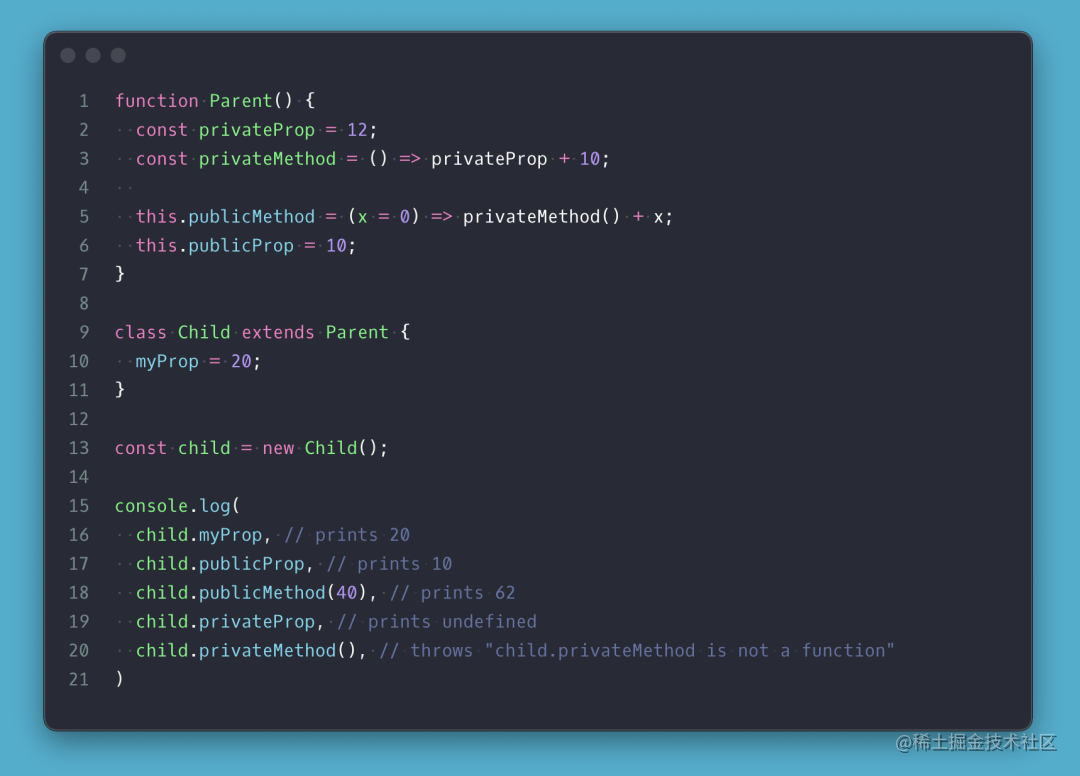## 20. 扩展构造函数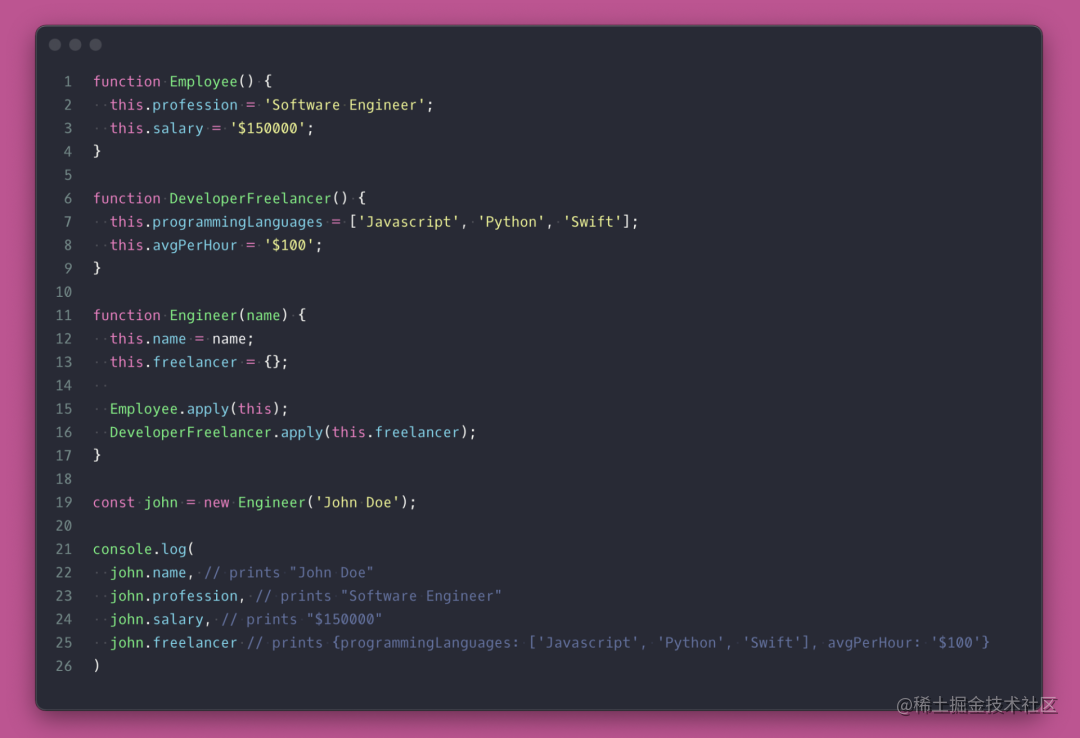## 21. 循环任何内容有时，你需要循环任何可迭代的内容（Set、Map、Object、Array、String 等）。这个非常简单的 forEach 函数工具就可以做到这一点。如果回调返回 true，它将退出循环。

``````function forEach(list, callback) {
const entries = Object.entries(list);
let i = 0;
const len = entries.length;
for(;i < len; i++) {
const res = callback(entries[i], entries[i], list);
if(res === true) break;
}
}

## 22. 使函数参数为 required

``````function required(argName = 'param') {
throw new Error(`"\${argName}" is required`)
}
function iHaveRequiredOptions(arg1 = required('arg1'), arg2 = 10) {
console.log(arg1, arg2)
}
iHaveRequiredOptions(); // throws "arg1" is required
iHaveRequiredOptions(12); // prints 12, 10
iHaveRequiredOptions(12, 24); // prints 12, 24
iHaveRequiredOptions(undefined, 24); // throws "arg1" is required

## 23. 创建模块或单例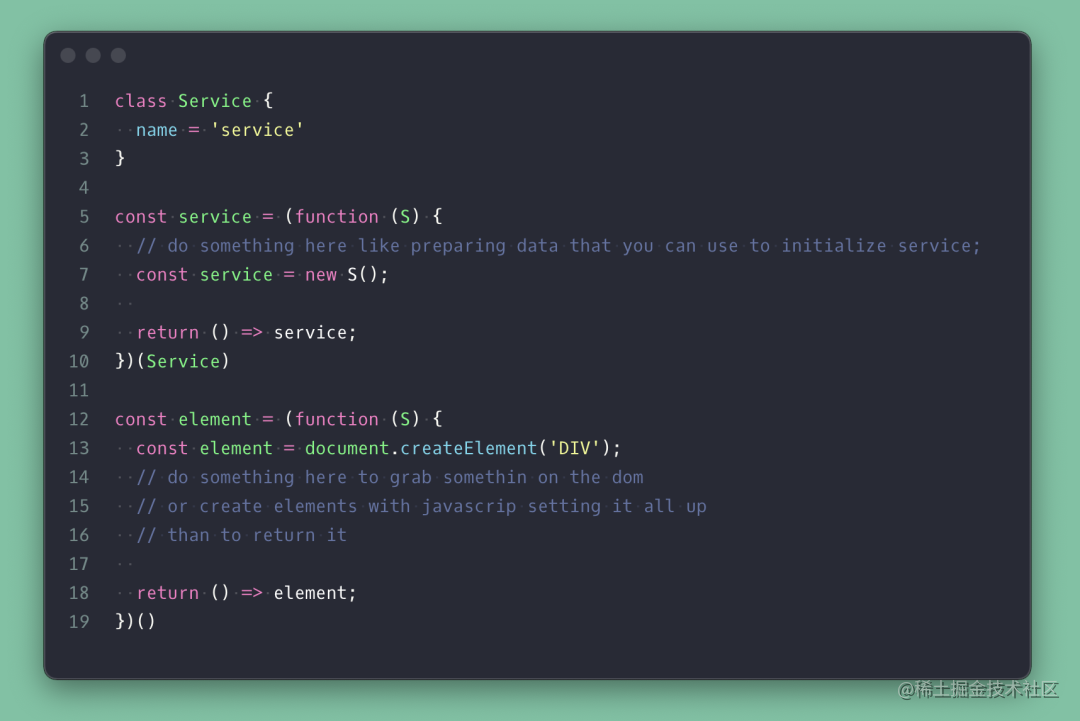## 24. 深度克隆对象开发人员通常会安装一些类似“lodash”的库来执行这一操作，但使用纯 JavaScript 来实现确实也很容易。这是一个简单的递归函数：只要是一个对象，就使用函数的构造器将其重新初始化为一个克隆，然后对所有属性重复该过程。

``````const deepClone = obj => {
let clone = obj;
if (obj && typeof obj === "object") {
clone = new obj.constructor();
Object.getOwnPropertyNames(obj).forEach(
prop => (clone[prop] = deepClone(obj[prop]))
);
}
return clone;
};

## 25. 深度冻结对象

``````const deepClone = obj => {
let clone = obj;
if (obj && typeof obj === "object") {
clone = new obj.constructor();
Object.getOwnPropertyNames(obj).forEach(
prop => (clone[prop] = deepClone(obj[prop]))
);
}
return clone;
};